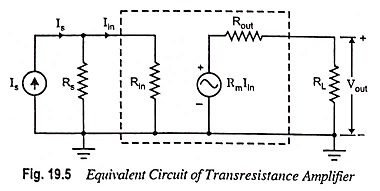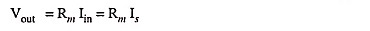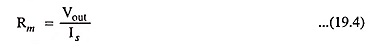## Transresistance Amplifier – Definition and Equivalent Circuit:

In transresistance amplifiers the input signal is current and the output signal is voltage. The ideal transresistance amplifier provides an output voltage in proportion to the signal current, and the proportionality constant is independent of the magnitudes of Rs and RL.From the equivalent circuit of a transresistance amplifier shown in Fig. 19.5, we find that if Rin << Rs then Iin ≈ Is and if Rout << RL, thenandAn ideal transresistance amplifier should have zero input and output resistances.

Scroll to Top